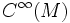Lie algebra of global derivations

• It has the structure of a$\R$-vector space under pointwise addition and scalar multiplication; more generally, it is a module over$C^\infty(M)$, the algebra of infinitely differentiable functions, under left multiplication
• It is a Lie algebra: the Lie bracket of two derivations$D_1$ and$D_2$ is given as:$D_1 \circ D_2 - D_2 \circ D_1$